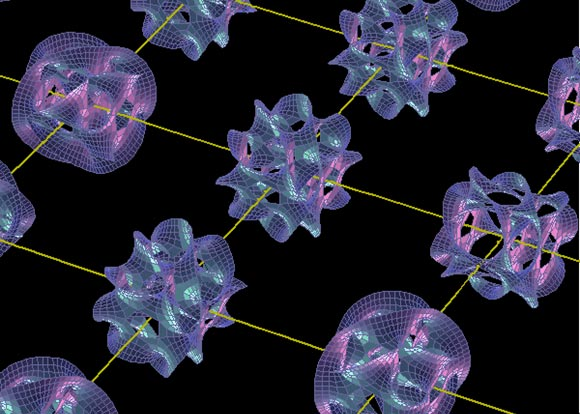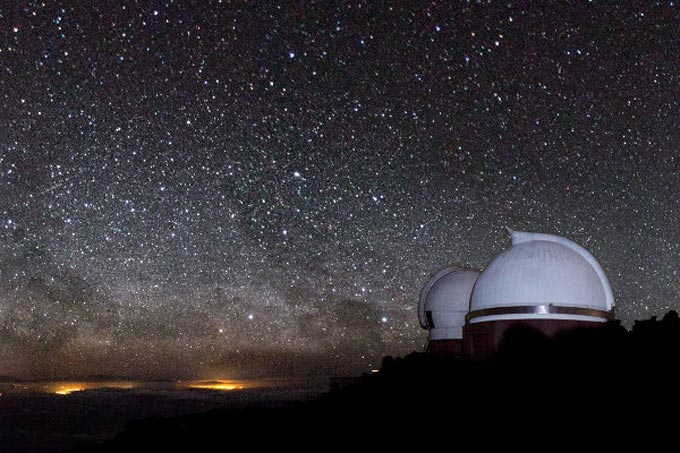Can It Be the Theory of Everything? | About Islam
Home > Science > Science & Tech > Can It Be the Theory of Everything?

Can It Be the Theory of Everything?Animation showing Quarks’ vibrations in hyper dimensions – String Theory.

Would you believe me if I told you that there is one unified master equation that can describe everything, every force in this world, every phenomenon in our cosmos, and how our universe was formed?

From atoms to galaxies?

Currently, this theory is just a proved complicated mathematical equation; however, scientists now are trying to prove it practically by one of the biggest experiments in science history.

Unifying Physics Laws in One Equation

The story began with a dream when Albert Einstein wanted to understand how the universe was formed. He followed his research on General Relativity by working to unify the General Theory of Relativity with electromagnetism in one equation. He spent the following 20 years trying to achieve this dream until his death.

What is the String Theory? Where are the 11-Dimentions?

As we already know there are three dimensions around us and we can feel them. Even though, it seemed to scientists that humanity cannot understand our universe with these three dimensions only, so we have to find out if there are other invisible dimensions around in this cosmos which could give us a deeper understanding of it.

Last century, Einstein spent a lot of time thinking about the issue and was able to come up with the Fourth Dimension, “Time”.

Nowadays, the four dimensions are all reasonable for human imagination. But we still don’t have a clear clue of how the universe was formed. The most reasonable answer for that question was the “Big Bang Theory” which proved that the universe started by a nuclear explosion of extremely tiny particles which are smaller than atoms called “Quarks”.

Quarks are zero dimensional but they vibrate in higher dimensions. What does that mean?This simply means that these very small quark particles vibrate like strings in a symphony.

From the largest Galactic Filament to the smallest particles of atoms and quarks, there is a continuous motion. Strings inside quarks vibrate in hyper-dimensions. String Theory aka M-Theory proved mathematically and theoretically that everything from atoms to galaxies vibrate in eleven invisible multi-dimensions.

To understand the universe, scientists had to assume that there are other parallel invisible dimensions, folded over each other.

Quantum Mechanics is of the most important branches in physics. It studies the motion of the very small things like electrons and atoms. That’s opposite to Einstein’s General Relativity which studies the motion of huge things like planets and galaxies.

The issue here was that when we use quantum mechanics to study the motion of planets, physics laws fully collapse since we use QM with the big scale. On the other hand, the general relativity can’t describe the motion of electrons within an atom. And that was a great problem.

How is the universe made up of tiny quarks while it can expand to become as widely big as it is right now; laws of physics must not contradict each other!

That’s why physicists tried to unify these two different theories and another two theories together into one master theory that describes everything to understand the universe and how it formed.

Wherefore scientists assumed other dimensions to get the master theory, and when they did that mathematically they found another equation from that assumption which helped them realize there are other dimensions and may be other “Parallel Universes”.

Do Multi-dimensions Really Exist?

Till 1954 this topic was only mathematically proved, thus physicists tried to prove it practically by establishing the world’s biggest laboratory for particle physics.

This big laboratory is the famous European Organization for Nuclear Research (CERN). About 2,600 people work there fulltime in addition to more than 7,800 scientists from about 500 universities and research institutes.

CERN is simply a particle accelerator in which particles get accelerated to reach the speed of light in an underground 27 km-long circular tunnel and then hit each other simulating the Big Bang cosmic event.

After the collision we study the energy emitted from every particle, calculations show that some particles’ energies disappear in what we call “parallel dimensions”. By detecting that disappearance of energy quantitatively we can prove the practical existence of hyper dimensions.

Nowadays there are results that may lead to prove observationally that there are many other dimensions in this universe. Let’s wait and see what tomorrow brings.

Finally, I recommend you to watch that amazing Ted-Talk “Making sense of string theory with Brian Greene: https://www.youtube.com/watch?v=YtdE662eY_M

This article was first published in 2015 and is currently republished for its importance.